## Posted tagged ‘fluid flow’

### 12. Energy Exchange with Moving Blades

September 22, 2011

#Subsections

• 12.1 Introduction
• 12.2 Conservation of Angular Momentum
• 12.3 The Euler Turbine Equation
• 12.4 Multistage Axial Compressors
• 12.5 Velocity Triangles for an Axial Compressor Stage
• 12.6 Velocity Triangles for an Axial Flow Turbine Stage

# 12.1 Introduction

So far we have only looked at the thermodynamic results of compressors and turbines (‘s and‘s). Now we will look in more detail at how the components of a gas turbine engine produce these effects. You will learn later that without heat transfer, it is only possible to change the total enthalpy of a fluid with an unsteady process (e.g. moving blades). Still we will use many of the steady flow tools that we have discussed in thermodynamics and propulsion by considering the steady flow in and out of a component as shown in Figure 12.1.# 12.2 Conservation of Angular Momentum

[This section is excerpted from Fluid Flow: A First Course in Fluid Mechanics, Macmillan Publishing Company, 1989.]

The momentum theorem developed in Chapter 10 gives the force acting on a fixed volume in terms of linear momentum flux through the surface of the volume. In many situations we are interested in the moment or torque on the volume. For this purpose we may adapt the angular momentum law of mechanics to the flow of fluids. Our starting point is the familiar law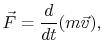where,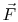, andrefer to a single particle. The torque exerted by the forceabout a fixed point iswhereis the radius vector from the fixed point to the point of application of. The symbol,, signifies, as usual, that the vector cross-product shall be taken. Then, from Newton’s law of motion,We now define a vectoras the vector product of the radius vector to the particle and the linear momentum, that is,The quantityis called angular momentum. Upon differentiatingwith respect to time, we find thatHowever,and the cross-product of a vector parallel to itself is zero. The first term in the right-hand side therefore vanishes and we have the result that(12..1)

Equation (12.1) states that the rate of change of angular momentum of a particle about a fixed point is equal to the torque applied to the particle.We now seek to modify the law as expressed by Equation (12.1) to be suitable for a fixed volume. The torque on a material volume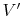is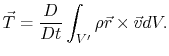This is readily transformed into a control volume integral. We have, therefore,where(12..2)

is the angular momentum contained within the control volume. Equation (12.2) represents the angular momentum theorem. [For more information about angular momentum and rotational energy, see pages 246 and 558 in Hibbeler’s Engineering Dynamics.]

# 12.3 The Euler Turbine Equation

The Euler turbine equation relates the power added to or removed from the flow, to characteristics of a rotating blade row. The equation is based on the concepts of conservation of angular momentum and conservation of energy. We will work with the model of the blade row shown in Figure 12.2.Applying conservation of angular momentum, we note that the torque,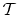, must be equal to the time rate of change of angular momentum in a streamtube that flows through the deviceThis is true whether the blade row is rotating or not. The sign matters (i.e. angular momentum is a vector – positive means it is spinning in one direction, negative means it is spinning in the other direction). So depending on how things are defined, there can be positive and negative torques, and positive and negative angular momentum. In Figure 9.2, torque is positive when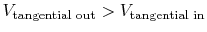V_{textrm{tangential in}}\$” width=”207″ height=”32″ align=”MIDDLE” border=”0″ /> — the same sense as the angular velocity.If the blade row is moving, then work is done on/by the fluid. The work per unit time, or power,, is the torque multiplied by the angular velocity,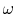:If torque and angular velocity are of like sign, work is being done on the fluid (a compressor). If torque and angular velocity are of opposite sign work is being extracted from the fluid (a turbine). Here is another approach to the same idea:

• If the tangential velocity increases across a blade row (where positive tangential velocity is defined in the same direction as the rotor motion) then work is added to the flow (this happens in a compressor).
• If the tangential velocity decreases across a blade row (where positive tangential velocity is defined in the same direction as the rotor motion) then work is removed from the flow (this happens in a turbine).

From the steady flow energy equation,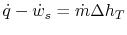with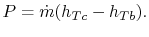Then equating this expression of conservation of energy with our expression from conservation of angular momentum, we arrive at:or for a perfect gas with,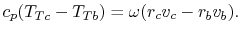(12..3)

Equation (12.3) is called the Euler Turbine Equation. It relates the temperature ratio (and hence the pressure ratio) across a turbine or compressor to the rotational speed and the change in momentum per unit mass. Note that the velocities used in this equation are what we will later call absolute frame velocities (as opposed to relative frame velocities).

• If angular momentum increases across a blade row, thenT_{Tb}\$” width=”80″ height=”32″ align=”MIDDLE” border=”0″ /> and work was done on the fluid (a compressor).
• If angular momentum decreases across a blade row, then <img src="http://web.mit.edu/16.unified/www/SPRING/propulsion/notes/img1569.png&quot; alt="\$ T_{Tc}  and work was done by the fluid (a turbine).

# 12.4 Multistage Axial Compressors

An axial compressor is typically made up of many alternating rows of rotating and stationary blades called rotors and stators, respectively, as shown in Figures 12.3 and 12.4. The first stationary row (which comes in front of the rotor) is typically called the inlet guide vanes or IGV. Each successive rotor-stator pair is called a compressor stage. Hence compressors with many blade rows are termed multistage compressors.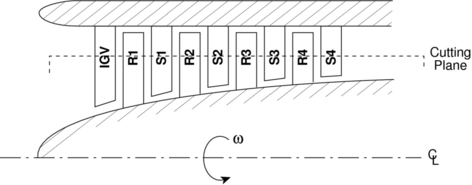One way to understand the workings of a compressor is to consider energy exchanges. We can get an approximate picture of this using the Bernoulli Equation, whereis the stagnation pressure, a measure of the total energy carried in the flow,is the static pressure, a measure of the internal energy, and the velocity terms are a measure of the kinetic energy associated with each component of velocity (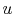is radial,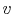is tangential,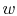is axial).The rotor adds swirl to the flow, thus increasing the total energy carried in the flow by increasing the angular momentum (adding to the kinetic energy associated with the tangential or swirl velocity,).The stator removes swirl from the flow, but it is not a moving blade row and thus cannot add any net energy to the flow. Rather, the stator rather converts the kinetic energy associated with swirl to internal energy (raising the static pressure of the flow). Thus typical velocity and pressure profiles through a multistage axial compressor look like those shown in Figure 12.5.Note that the IGV also adds no energy to the flow. It is designed to add swirl in the direction of rotor motion to lower the Mach number of the flow relative to the rotor blades, and thus improve the aerodynamic performance of the rotor.

# 12.5 Velocity Triangles for an Axial Compressor Stage

Velocity triangles are typically used to relate the flow properties and blade design parameters in the relative frame (rotating with the moving blades), to the properties in the stationary or absolute frame.

We begin by “unwrapping” the compressor. That is, we take a cutting plane at a particular radius (e.g. as shown in Figure 12.4) and unwrap it azimuthally to arrive at the diagrams shown in Figure 12.6. Here we have assumed that the area of the annulus through which the flow passes is nearly constant and the density changes are small so that the axial velocity is approximately constant.In drawing these velocity diagrams it is important to note that the flow typically leaves the trailing edges of the blades at approximately the trailing edge angle in the coordinate frame attached to the blade (i.e. relative frame for the rotor, absolute frame for the stator).

We will now write the Euler Turbine Equation in terms of stage design parameters:, the rotational speed, and, the leaving angles of the blades.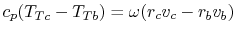From geometry,and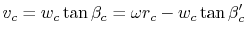soorSo we see that the total or stagnation temperature rise across the stage increases with the tip Mach number squared, and for fixed positive blade angles, decreases with increasing mass flow. This behavior is represented schematically in Figure 12.7.# 12.6 Velocity Triangles for an Axial Flow Turbine Stage

We can apply the same analysis techniques to a turbine, Figure 12.8. The stator, again does no work. It adds swirl to the flow, converting internal energy into kinetic energy. The turbine rotor then extracts work from the flow by removing the kinetic energy associated with the swirl velocity.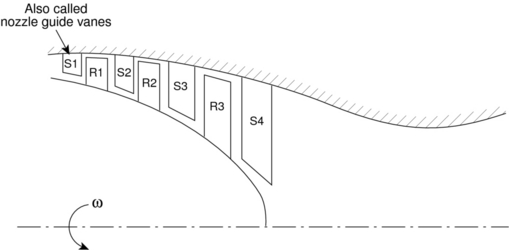The appropriate velocity triangles are shown in Figure 12.9, where again the axial velocity was assumed to be constant for purposes of illustration. As we did for the compressor, we can write the Euler Turbine Equation in terms of useful design variables:### Fluid Machines – Pumps and Turbines

September 18, 2011What is a fluid Machine ? A fluid machine is a device which converts the energy stored by a fluid in to mechanical energy or work.   What are positive displacement machines ? The machine functioning depend essentially on the change of volume of a certain amount of fluid within the machine. There is a physical displacement of the boundary of certain fluid hence it is called positive displacement machine.  Based on the direction of fluid flow how are the fluid machines classified ? According to the flow direction of fluid, the fluid machines are classified as Axial flow machines – The main flow direction is parallel to the axis of the machine. Radial flow machines – The main flow direction is perpendicular to the axis of the machine ie. in radial direction Mixed flow machines – The fluid enters radially and leaves axially or vice-versa.  What is a turbine ? A turbine converts the energy of the fluid in to mechanical energy which is then utilized in running a generator of a power plant.  State the difference between impulse and reaction turbines ? At the inlet of the turbine the energy available is only kinetic energy, the turbine is known as impulse turbine. If the inlet of the turbine, possesses kinetic energy and pressure energy, the turbine known as reaction turbine.  What are Homologous units ? In utilizing scale models in designing turbo machines, geometric simililitude is necessary as well as geometrically similar velocity diagrams at entrance and exit from impellers. Two geometrically similar units having similar vector diagrams are called homologous units.  Define specific speed based on power. The specific speed (Ns ) of turbine is defined as the speed of some unit of series of such size that it produces unit power with unit head. .  Define specific speed based on discharge. The specific speed ( Ns ) of the pump is defined as the speed of some unit of the series of such size that it delivers unit discharge at unit speed  What is the significance of specific speed ? The specific speed inversely proportional to the head across the machine. So low specific speed corresponds to high head across it and vice-versa. The specific speed is directly proportional to the discharge through the machine or power produced by the machine. So low specific speed therefore refers to low discharge or low power machine and vice-versa.  Name the main parts of a radial flow reaction turbine ? i) Casing ii) Guide vane iii) Runner iv) Draft tube.  What is the purpose of the guide vanes in radial flow reaction turbine ? The purpose of the guide vane is to convert a part of pressure energy of the fluid at its entrance to the kinetic energy and then direct the fluid on to the number of blades.  What is a draft tube ? In which turbine it is mostly used. The draft tube is a conduit which connects the runner exit to the trail race, when water is being finally discharged from the turbine. In reaction turbine , the draft tube is mostly used.  What is the primary function of a draft tube ? The primary function of the draft tube is to reduce the velocity of the discharged water to minimize the loss of kinetic energy at the outlet.  Name the main parts of Kaplan turbine. i). Scroll casing ii). Guide vanes iii). Hub with vanes iv). draft tube  Name the main parts of pelton wheel . i) Nozzle ii) Runner with buckets iii) Casing.  An inward flow reaction turbine running at 250rpm has D1 = 1m, b1 = 0.2m, D2 = 0.5m and b3 = 0.3m. If the water enters the wheel radially at 3.5m/s velocity, determine the discharge and velocity of the flow at the outlet. Assume vane thickness at the extremities to be negligible. Q = p D1 b1 Uf1 = p D2 b2 Uf2 = p x 1 x 0.2 x 3.5 = 2.199 m3/s Uf2 = 2.99/ (p x 0.5 x 0.3) = 4.666 m/s  What are the different performance characteristic curves ? i) Variable speed curves (or) main characteristics ii) Constant seed curves (or) operating characteristics iii) Constant efficiency curves (or) Muschel characteristics  Give an example for a low head turbine , a medium head turbine and a high head turbine. Low head turbine – Kaplan turbine Medium head turbine – Francis turbine High head turbine – Pelton wheel.  what are the purposes of casing of a centrifugal pump i) To provide water to and from the impeller ii) To partially convert the kinetic energy in to pressure energy  What are the different types of casing in centrifugal pump ? i) Volute casing ii) Turbine casing (or) casing with guide blades  What is a positive displacement pump ? In the case of positive displacement pumps, the fluid is physically pushed from an enclosed space. The positive displacement pumps can be either reciprocating type or rotary type.  Where is the reciprocating pump well suited ?> The reciprocating pump is well suited for relatively small capacities and high heads.  what are the main components of a reciprocating pump ? 1)cylinder 2) Piston 3) suction valve 4) Delivery valve 5) Suction pipe 6) delivery pipe 7) crank shaft and connecting rod mechanism.  Define coefficient of discharge of reciprocating pump. The ratio between the actual discharge and theoretical discharge is known as coefficient of discharge. Cd = Qact / Qthe.  Define slip of the reciprocating pump. When does the negative slip occurs ? The difference between theoretical discharge and actual discharge is called the slip of the pump. Slip = Qthe. – Qact , % of slip = (Qthe. – Qact ) x 100 / Qthe. Negative slip occurs when delivery pipe is short suction pipe is long and pump is running at high speed.  Differentiate between single acting and double acting reciprocating reciprocating pump. In single acting pump, there is one suction valve and one delivery valve. On the backward stroke of the piston, the suction valve opens and water enters into the cylinder space. On the forward stroke, the suction valve closes and delivery valve opens, the water is forced through the delivery pipe. In the double acting pump, there are two suction valves and two delivery vales one in the front and one in the rear. When the piston moves backward, the suction valve in the front opens and delivery valve in the rear opens and water is forced through it. When the piston moves forward, the suction valve in the rear opens and delivery valve in the front opens and water is forced through it.  What is an indicator diagram of a reciprocating pump ? The indicator diagram of a reciprocating pump is the diagram which shows the pressure head in the cylinder corresponding to any position during the suction and delivery strokes.  A single acting single cylinder reciprocating pump has the following characteristics. Discharge = 6 lps, suction head = 4m, Delivery head = 20m, Find the energy required to drive the pump. Energy required = r g Q ( Hs + Hd ) Watts = (1000 x9.81 x 6×10-3 x (4 + 20) / 1000 = 1.409 kW.  What is an air vessel and what is its purpose ? An air vessel is a closed chamber connected on the suction or delivery or both sides of the reciprocating pump to obtain a more uniform flow.  Name some rotary positive displacement pumps. a. Gear pumps. b. Vane Pumps. c. Piston pumps. d. Screw pumps.